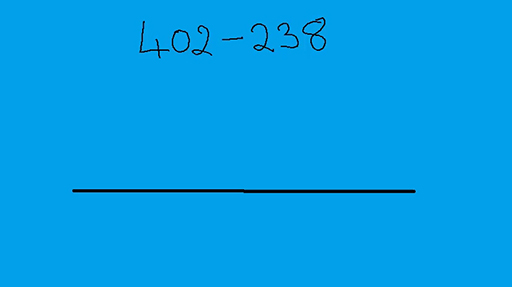Teaching mathematics

Start this free course now. Just create an account and sign in. Enrol and complete the course for a free statement of participation or digital badge if available.

Free course

# 2.2 Mental addition and subtraction strategies

Using number bonds can help with mental addition calculations because numbers can be split up to make the tens or hundreds number and then the extra can be added on.

## Activity 3 Adding large numbers mentally

Timing: Allow 5 minutes

Try these questions and reflect on your methods.

• a.

• b.

• c.

### Discussion

Did you partition the numbers into smaller numbers? For example, for question (a) did you work out 40 + 30 and then work out 3 + 2?

Did you bridge across a multiple of ten? For example, in question (b) did you mentally change 47 + 38 into 47 + 3 (to make 50) and then add 35?

Once a learner can calculate what to add to 38 to make 100 they can answer the question 100 – 38 and move on to calculations such as 402 – 238 by bridging through 300.

This mental arithmetic can be illustrated using an empty number line. The actions on the number line can act as a way to record the stages in a mental calculation. This is shown in the following video.

Download this video clip.Video player: Video 1 Using the empty number line to subtract 238 from 402Skip transcript: Video 1 Using the empty number line to subtract 238 from 402

#### Transcript: Video 1 Using the empty number line to subtract 238 from 402

INSTRUCTOR:
I'm going to calculate 402 takeaway 238 using the number line. And I've drawn a number line ready for this below. The way I'm going to do this is to actually work out what needs to be added to 238 to make 402.
So I'll start with 238, and I'll end with 402. This is a process known as complementary addition. First of all, I'm going to go to the first tens number after 238-- so that's 240. And to do that, I shall have added 2.
Next I'll work up to the next hundreds number. That's 300. And to make that jump, I should have added 60. And finally, I can get from 300 to 402 in one jump by adding 102. So altogether, I will have added 102, 60, and 2, and that is 164. So 402 take 238 is 164.
End transcript: Video 1 Using the empty number line to subtract 238 from 402
Video 1 Using the empty number line to subtract 238 from 402
Interactive feature not available in single page view (see it in standard view).

We can use this method to answer questions such as £4.02 – £2.38 or 4.03 m – 2.38 m, taking us into the realm of using measures. This method also helps with time calculations where learners need to remember that time is not a decimal system. For example, a train journey which starts at 2.38 pm and goes on until 4.02 pm takes 22 minutes + 1 hour + 2 minutes which comes to 1 hour 24 minutes (bridging through 3 pm and 4 pm).

## Activity 4 Subtraction problems for you to solve

Timing: Allow 5 minutes

• a.

• b.

• c.

TM_1